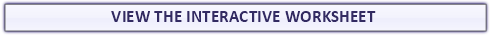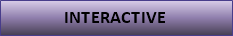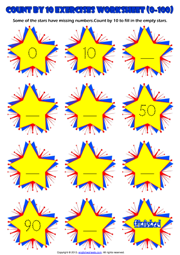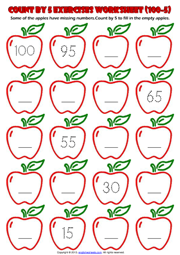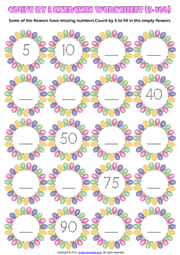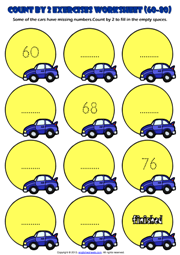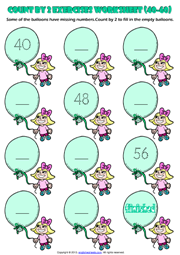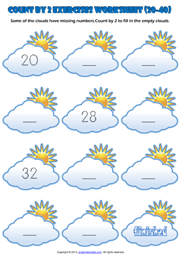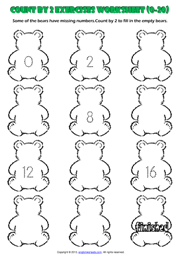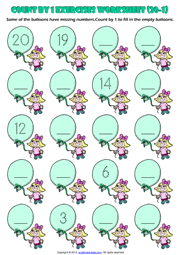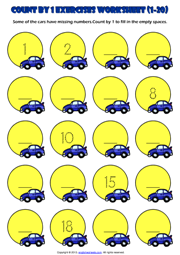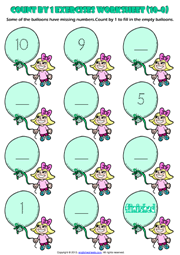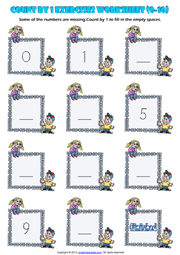# Counting Numbers Printable Maths Worksheets and Exercises

Here on this page you can download and use Printable Grade 1 Maths Worksheets, Exercises, Handouts, Tests, Activities, Teaching and Learning Resources, Materials  for kids about Counting Numbers by 1, 2, 5 and 10

## Counting Forward by 1 from 0 to 10 Exercises Worksheet

Counting Forward Exercises Maths Worksheet for grade 1 (first grade) students and kids with boy and girl theme to practise counting from 1 to 10. Some of the numbers in the pictures are missing. Count by 1 (one) and fill in the empty spaces. (from zero to ten)

## Counting Backwards by 1 from 10 to 0 Exercises Worksheet

Counting Backwards Exercises Maths Worksheet for grade 1 (first grade) students and kids with balloon and girl theme to practise counting from 10 to 0. Some of the numbers in the balloons are missing. Count by 1 (one) and fill in the empty balloons. (from ten to zero)

## Counting Forward by 1 from 1 to 20 Exercises Worksheet

Counting Forward Exercises Maths Worksheet for grade 1 (first grade) students and kids with blue cars theme to practise counting by 1 from 1 to 20. Some of the cars have missing numbers. Count by 1 (one) and fill in the empty spaces. (from one to twenty)

## Counting Backwards by 1 from 20 to 1 Exercises Worksheet

Counting Backwards Exercises Maths Worksheet for grade 1 (first grade) students and kids with balloon and girl theme to practise counting by 1 from 20 to 1. Some of the cars have missing numbers. Count by 1 (one) and fill in the empty spaces. (from twenty to one)

## Counting Forward by 2 from 0 to 20 Exercises Worksheet

Counting Exercises Maths Worksheet for grade 1 (first grade) students and kids with bears theme to practise counting forward by 2 from 0 to 20. Some of the numbers in the bears are missing. Count by 2 (two) and fill in the empty spaces. (from zero to twenty)

## Counting Forward by 2 from 20 to 40 Exercises Worksheet

Counting Exercises Maths Worksheet for grade 1 (first grade) students and kids with clouds and sun theme to practise counting forward by 2 from 20 to 40. Some of the numbers in the clouds are missing. Count by 2 (two) and fill in the empty clouds. (from twenty to forty)

## Counting Forward by 2 from 40 to 60 Exercises Worksheet

Counting Exercises Maths Worksheet for grade 1 (first grade) students and kids with balloon and girl theme to practise counting forward by 2 from 40 to 60. Some of the balloons have missing numbers. Count by 2 (two) and fill in the empty spaces. (from forty to sixty)

## Counting Forward by 2 from 60 to 80 Exercises Worksheet

Counting Exercises Maths Worksheet for grade 1 (first grade) students and kids with blue cars theme to practise counting forward by 2 from 60 to 80. Some of the cars have missing numbers. Count by 2 (two) and fill in the empty spaces. (from sixty to eighty)

## Counting Forward by 5 from 5 to 100 Exercise Worksheet

Counting Exercise Maths Worksheet for grade 1 (first grade) students and kids with abstract flowers theme to practise counting forward by 5 from 0 to 100. Some of the numbers in the flowers are missing. Count by 5 (five) and fill in the empty flowers. (from five to one hundred)

## Counting Backwards by 5 from 100 to 5 Exercise Worksheet

Counting Exercise Maths Worksheet for grade 1 (first grade) students and kids with red apples theme to practise counting backwards by 5 from 100 to 5. Some of the numbers in the apples are missing. Count back by 5 (five) and fill in the empty apples. (from one hundred to five)

## Counting Forward by 10 from 0 to 100 Exercise Worksheet

Counting Exercise Maths Worksheet for grade 1 (first grade) students and kids with yellow stars theme to practise counting forward by 10 from 0 to 100. Some of the stars have missing numbers. Count by 10 (ten) and fill in the empty stars. (from zero to one hundred)

## Counting Backwards by 10 from 100 to 0 Exercise Worksheet

Counting Exercise Maths Worksheet for grade 1 (first grade) students and kids with lorries theme to practise counting backwards by 10 from 100 to 0. Some of the lorries have missing numbers. Count by 10 (ten) and fill in the empty lorries. (from one hundred to zero)